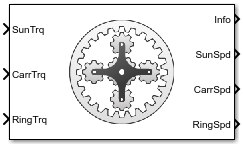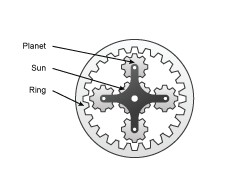# Planetary Gear

Ideal planetary gear with sun, ring, and carrier

• Library:
• Powertrain Blockset / Drivetrain / Couplings

•## Description

The Planetary Gear block implements an ideal planetary gear coupling consisting of a rigidly coupled sun, ring, and carrier gears. The block calculates the dynamic response to the sun, carrier, and ring input torques.In fuel economy and powertrain studies, you can use the Planetary Gear block as a power-split device by coupling it to common driveline elements such as transmissions, engines, clutches, and differentials.

These equations of motion represent the dynamic response of the planetary gear.

`$\begin{array}{l}{\stackrel{˙}{\omega }}_{s}{J}_{s}={\stackrel{˙}{\omega }}_{s}{b}_{s}+{T}_{s}+{T}_{ps}\\ {\stackrel{˙}{\omega }}_{c}{J}_{c}={\stackrel{˙}{\omega }}_{c}{b}_{c}+{T}_{c}+{T}_{pc}\\ {\stackrel{˙}{\omega }}_{s}{J}_{r}={\stackrel{˙}{\omega }}_{r}{b}_{r}+{T}_{r}+{T}_{pr}\\ {\stackrel{˙}{\omega }}_{p}{J}_{p}={\omega }_{p}{b}_{p}+{T}_{rp}+{T}_{sp}+{T}_{cp}\end{array}$`

To reduce the equations of motion, the block uses these kinematic and geometric constraints.

`$\begin{array}{l}{\omega }_{c}{r}_{c}={r}_{s}{\omega }_{s}+{r}_{p}{\omega }_{p}\\ {\omega }_{r}{r}_{r}={r}_{c}{\omega }_{c}+{r}_{p}{\omega }_{p}\\ {r}_{c}={r}_{s}+{r}_{p}\\ {r}_{r}={r}_{c}+{r}_{p}\end{array}$`

### Power Accounting

For the power accounting, the block implements these equations.

Bus Signal DescriptionEquations

`PwrInfo`

`PwrTrnsfrd` — Power transferred between blocks

• Positive signals indicate flow into block

• Negative signals indicate flow out of block

`PwrSun`

Sun gear applied power

${\omega }_{s}{T}_{s}$

`PwrCarr`Carrier gear applied power

${\omega }_{c}{T}_{c}$

`PwrRing`Ring gear applied power

${\omega }_{r}{T}_{r}$

`PwrNotTrnsfrd` — Power crossing the block boundary, but not transferred

• Positive signals indicate an input

• Negative signals indicate a loss

`PwrDampLoss`Mechanical damping loss

$-\left({b}_{s}{\omega }_{s}^{2}+{b}_{c}{\omega }_{c}^{2}+{b}_{r}{\omega }_{r}^{2}+{b}_{p}{\omega }_{p}^{2}\right)$

`PwrStored` — Stored energy rate of change

• Positive signals indicate an increase

• Negative signals indicate a decrease

`PwrStoredPlntry`

Rate change in rotational kinetic energy

The equations use these variables.

 ωc, ωp, ωr, ωs Carrier, planet, ring, and sun gear angular speed rc, rp, rr, rs Carrier, planet, ring, and sun gear angular radius Jc, Jp, Jr, Js Carrier, planet, ring, and sun gear inertia bc, bp, br, bs Carrier, planet, ring, and sun gear damping Tc, Tp, Tr, Ts Applied carrier, planet, ring, and sun gear torque Tps Torque applied from planet gear on sun gear Tpc Torque applied from planet gear on carrier gear Tpr Torque applied from planet gear on ring gear Trp Torque applied from ring gear on planet gear Tsp Torque applied from sun gear on planet gear Tcp Torque applied from carrier gear on planet gear

## Ports

### Input

expand all

Sun gear input torque, Ts, in N·m.

#### Dependencies

To create this port, for Port Configuration, select `Simulink`.

Carrier gear input torque, Tc, in N·m.

#### Dependencies

To create this port, for Port Configuration, select `Simulink`.

Ring gear applied torque, Tr, in N·m.

#### Dependencies

To create this port, for Port Configuration, select `Simulink`.

Carrier gear angular speed, ωc, in rad/s. Carrier gear applied torque, Tc, in N·m.

#### Dependencies

To create this port, for Port Configuration, select `Two-way connection`.

### Output

expand all

Bus signal containing these block calculations.

SignalDescriptionUnits
Sun

`SunTrq`

Sun gear applied torque

N·m

`SunSpd`

Sun gear angular speed

Carr

`CarrTrq`

Carrier gear applied torque

N·m

`CarrSpd`

Carrier gear angular speed

Ring

`RingTrq`

Ring gear applied torque

N·m
PwrInfo

`PwrTrnsfrd`

`PwrSun`

Sun gear applied powerW
`PwrCarr`Carrier gear applied powerW
`PwrRing`Ring gear applied powerW

`PwrNotTrnsfrd`

`PwrDampLoss`Mechanical damping lossW

`PwrStored`

`PwrStoredPlntry`

Rate change in rotational kinetic energy

W

Sun gear angular speed, ωs, in rad/s.

#### Dependencies

To create this port, for Port Configuration, select `Simulink`.

Carrier gear angular speed, ωc, in rad/s.

#### Dependencies

To create this port, for Port Configuration, select `Simulink`.

Ring gear angular speed, ωr, in rad/s.

#### Dependencies

To create this port, for Port Configuration, select `Simulink`.

Sun gear angular speed, ωs, in rad/s. Sun gear applied torque, Ts, in N·m.

#### Dependencies

To create this port, for Port Configuration, select `Two-way connection`.

Ring gear angular speed, ωr, in rad/s. Ring gear applied torque, Tr, in N·m.

#### Dependencies

To create this port, for Port Configuration, select `Two-way connection`.

## Parameters

expand all

Block Options

Specify the port configuration.

#### Dependencies

Specifying `Simulink` creates these ports:

• `SunTrq`

• `CarrTrq`

• `RingTrq`

• `SunSpd`

• `CarrSpd`

• `RingSpd`

Specifying `Two-way connection` creates these ports:

• `C`

• `S`

• `R`

Sun-to-planet gear ratio, dimensionless.

Sun-to-ring gear ratio, dimensionless.

Sun gear inertia, Js, in kg·m^2.

Planet gear inertia, Jp, in kg·m^2.

Ring gear inertia, Jr, in kg·m^2.

Carrier gear inertia, Jc, in kg·m^2.

Sun gear viscous damping, bs, N·m· s/rad.

Ring gear viscous damping, br, N·m· s/rad.

Planet gear viscous damping, bp, N·m· s/rad.

Carrier gear viscous damping, bc, N·m· s/rad.

Initial sun gear angular speed, in rad/s.

Initial carrier gear angular speed, in rad/s.

## Version History

Introduced in R2017a